Checkout JEE MAINS 2022 Question Paper Analysis : Checkout JEE MAINS 2022 Question Paper Analysis :

# Construction Of Perpendicular Lines

Construction of Perpendicular Lines: When two lines intersect to form right angles then such lines are known as perpendicular to each other. Perpendicular lines lie in the same plane i.e. they are co-planar and intersect at right angles. Thus it implies that if you have two lines which are perpendicular to each other, then these lines will be at right angles and vice versa. With the help of ruler and compass we can easily construct a line perpendicular to another line and passing through a point given on that line.

Construction of Perpendicular Lines Brief

Before getting into the construction procedure, let us discuss the brief notes on the construction of the perpendicular lines. The first step to construct the line segment is that draw the line segment for the given measurement. Then mark the point on the line and place the compass on the given point. Now draw the arc across the given line on each side. Without adjusting the compass, draw another arc. It is noted that, whenever a perpendicular bisector is drawn for the given, the division of the line segment gives the equal measure on both the sides of the line should be equal.

## Steps to Construct Perpendicular lines

Constructing a perpendicular to a line through a point on it

Given: A line segment AB with a point P on it.

To construct A line perpendicular to line AB passing through point P.

Step 1: With P as center and any suitable radius, draw an arc cutting line segment AB at two distinct points as shown in the given figure.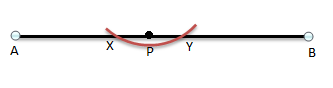Step 2: Now with X as center and a suitable radius draw an arc on either side of the given line segment AB. Also, with Y as center and same radius as previous, draw an arc on either side of the given line segment cutting the arc drawn through point X at M as shown in the given figure.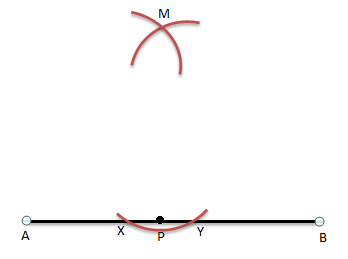Step 3: Join the point M and P as shown, and the line segment MP is the required perpendicular to AB through point P.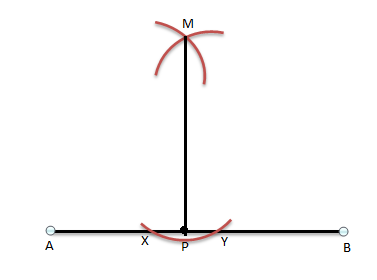Let us see if the line segment MP constructed is actually perpendicular to AB or not.

Join M to the points X and Y as shown: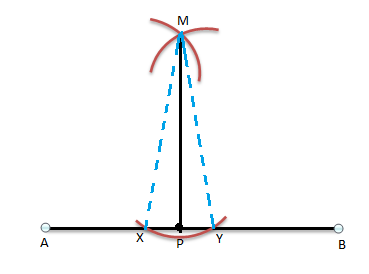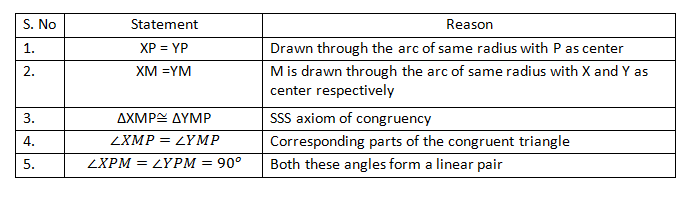Thus, the constructed line segment MP is perpendicular to AB. Using the above construction, we can easily construct two perpendicular lines using compass and a ruler.